##### Signals and Systems For DummiesVisualize the big picture of the AM radio transmitter, receiver, and interfering signals with a system block diagram. Each block in the diagram has an underlying mathematical model.

The signal model for an AM signal is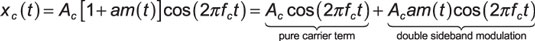where Ac is the carrier amplitude, fc is the carrier frequency, m(t) is the message signal, and the modulation index is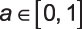The modulation index controls how much of the signal is made up of the message and how much is pure carrier.

The AM scheme also requires that min[m(t)] ≥ –1. A simple decomposition of xc(t) shows that a pure carrier term and a double sideband modulation term are involved. The term double sideband comes from viewing xc(t) in the frequency domain.

The second term follows from the modulation theorem for Fourier transforms (FT). For m(t) real, the magnitude spectrum |M(f)| is symmetrical about f = 0. Multiplying by cos(2πfct) shifts M(f) up and down in frequency by the carrier frequency fc.

The spectrum of M(ffc) just above fc is known as the upper sideband (USB), and the one just below fc is known as the lower sideband (LSB). The same relation holds for the M(f + fc) term. The USB and LSB contain the same information — hence the term double sideband.

The figure shows the frequency domain details of the AM signal.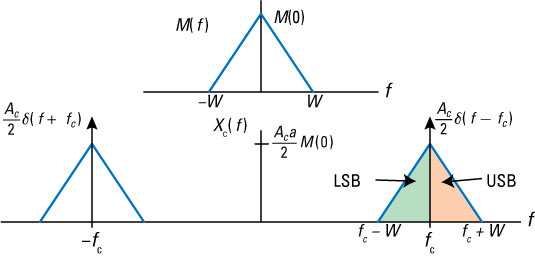Credit: Illustration by Mark Wickert, PhD

## Look at the waveform

The AM waveform, shownhere for a particular m(t), motivates a simple receiver design.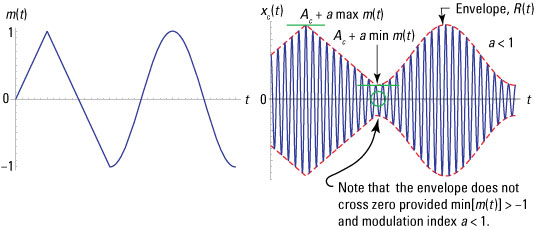Credit: Illustration by Mark Wickert, PhD

The envelope of xc(t) is the dashed line, given by R(t) = Ac(1 + am(t)). As long as min[am(t)] > –1, retaining just the positive half cycles of xc(t) allows R(t) to accurately contain m(t). In electronic circuit form, the recovery of m(t) from xc(t) is accomplished with an envelope detector as shown.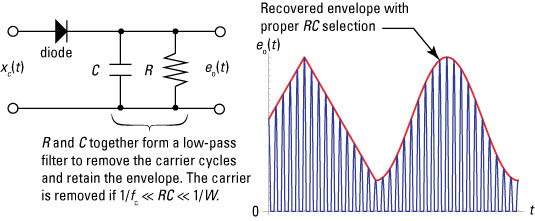Credit: Illustration by Mark Wickert, PhD

You need a direct current (DC) block or level shift to finally get a term proportional to m(t). Circuit theory tells you that a DC block can be as simple as placing a coupling capacitor in a series with the detector output and the next stage of signal processing, which may be an amplifier used to drive headphones or a speaker, for example.

## Complete the diagram

The system block diagram for the transmitter and receiver (transceiver) is shown here.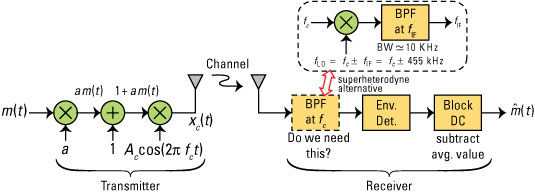Credit: Illustration by Mark Wickert, PhD

There's an additional component, namely a band-pass filter (BPF), in front of the envelope detector. The filter is expected to combat the adjacent channel interference to the receiver model. A tunable BPF filter centered at fc can become a BPF with center frequency fixed at fIF if you place a mixer (multiplier) in front of it.

Now you need a local oscillator (LO) to shift the frequency of the received signal from fc to fIF. The local oscillator signal is actually a cosine signal at frequency fc +/– fIF. The math behind the frequency shifting is based on the FT modulation theorem. The inclusion of the frequency shifting front-end is known as a superheterodyne receiver.